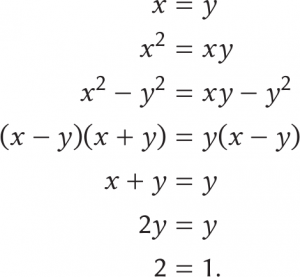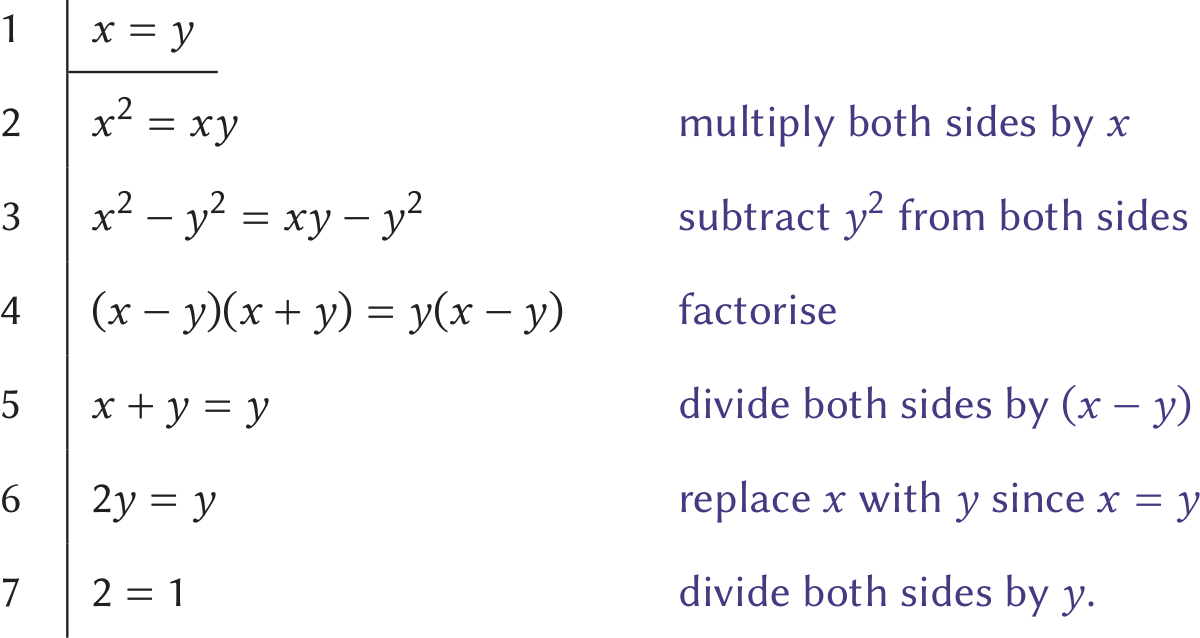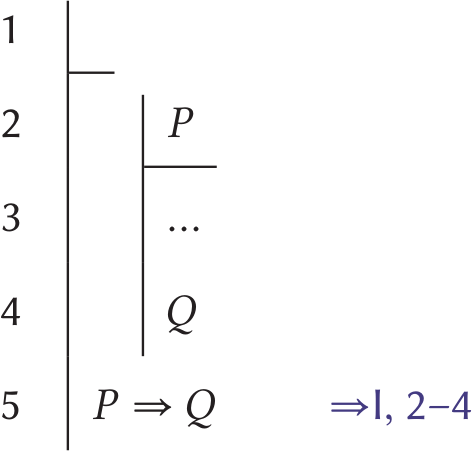# How can we tell that 2≠1?

Maynard manages to prove that 2≠1 in less space than it took Bertrand Russell to prove that 1+1=2What if I told you that $2=1$? You may say I’m wrong. OK, well, what if I proved it to you? We can both agree that there’s an $x$ and a $y$ where $x = y$. From there, multiply, subtract, factorise, divide, substitute, divide again, and you get $2 = 1$.

Still not happy? You’re probably unconvinced by my so-called ‘proof’. OK, I say, and, after a minute, hand you a sheet of paper with the following hastily scrawled on it:It’s better, but you’re still displeased. This time, I’ve made clear what steps I’m taking from $x = y$ to $2 = 1$. However, you point out, I don’t connect any of these steps. Nodding slowly, I take my time and write out a very nice, orderly proof, complete with justifications for each line:At this point, you spot my mistake: in going from line 4 to 5, I have divided both sides by $x-y$. But we began with the assumption that $x = y$, meaning that $x-y = 0$, and dividing by 0 is not defined! This means that lines 5 to 7 are operating on nonexistent values and are therefore meaningless.

You’re happy with yourself, but something is bothering you. To reveal my mistake, you asked me to be more precise. But why stop here? Because you found what you were looking for? That’s not how truth is found.

My proof, like all proofs, is a path from one statement to another, just as we may follow the path from $ax^2 + bx + c = 0$ to $x = \big(-b \pm \sqrt{b^2-4ac}\big)/{2a}$, or from the existence of rectangles to the transitivity of parallelism (see below). Along this path I have made several intermediate statements, and linked them together with justifications. You found that one of my links is flawed, and you wonder how we know that the others aren’t also wrong. You begin to question foundational principles, wondering, for instance, why we’re even allowed to do the same thing to both sides of an equation.

Euclidean geometry: For the unfamiliar—Euclidean geometry (standard geometry on a flat surface) rests on 5 assumptions, one of which (the parallel postulate) has historically been regarded as ugly. In attempting to eliminate the parallel postulate, mathematicians have found numerous other statements that are equivalent to it, such as that a rectangle exists or that parallelism is transitive.

You keep digging deeper and deeper, questioning more and more of what you previously took to be correct. Eventually, you come across a piece of mathematics that is perhaps the most beautiful and elegant thing you’ve ever laid your eyes upon: natural deduction.

## Natural deduction

Natural deduction is one result of asking for deeper and deeper justification when doing maths. A system of natural deduction is a set of very simple, almost irrefutable rules that act to formalise our intuition about what is definitely true.

These rules include such things as reiteration, which simply allows us to repeat ourselves. Precisely, reiteration says that if you know that a statement $P$ is true, then you can conclude that $P$ is true. This is hardly controversial.

There are two rules for the natural idea of ‘and’. First is the so-called conjunction introduction rule, stating that if you know that $P$ and $Q$ are both true, then you may conclude $P \land Q$, pronounced ‘$P$ and $Q$’. On the other side, we have conjunction elimination, stating that if you know that $P \land Q$ is true, then you may conclude $P$ and also may conclude $Q$.

These rules don’t feel like they do much besides swapping out ‘and’ for ‘$\land$’; however, doing so is important for formality and precision.

Things start to get tricky with the rules codifying ‘or’. The first, disjunction introduction, tells us that if $P$ is true, then you may conclude $P \lor Q$, pronounced ‘$P$ or $Q$’: if I am hungry, then it’s also true that I’m either hungry or tired.Disjunction elimination ($\lor$E): if $P\lor Q$ and from $P$ we can prove $X$ and from $Q$ we can prove $X$, then $X$.

The second rule, disjunction elimination, states that if $P \lor Q$ is true, and from $P$ you can prove $X$, and from $Q$ you can prove $X$, then you may conclude $X$. More colloquially, if either $P$ or $Q$ is true, and in both cases $X$ is true, too, then $X$ is true. For example, if I’m either well-rested or well-fed, and being well-rested makes me happy, and being well-fed makes me happy, then I must be happy.Implication introduction ($\Rightarrow$I): if from $P$ we can prove $Q$, then $P\Rightarrow Q$.

Then come the rules regarding implication. We have implication introduction, stating that if from $P$ we can prove $Q$, then we may conclude $P \Rightarrow Q$, pronounced ‘$P$ implies $Q$’. And we have implication elimination (also known as modus ponens), which states that if $P \Rightarrow Q$ is true and $P$ is true, then we can conclude $Q$. If the weather being rainy implies that I am cosy, and the weather is rainy, then I must be cosy.

Finally, we come to the most arcane rules, those handling negation. The negation of $P$ is written $\neg P$ and pronounced ‘not $P$’. Before talking about the $\neg P$ rules, however, we must first introduce a new symbol: $\bot$ (pronounced ‘bottom’), which represents impossibility or contradiction. We can then introduce bottom introduction, which states that if both $P$ and $\neg P$ are true, which is absurd (usually… there are systems of logic that admit both $P$ and $\neg P$ at the same time, called paraconsistent logics), then we can conclude $\bot$, to represent this impossibility.

We’re then able to make use of $\bot$ through negation introduction, which states that if from $P$ we can prove $\bot$, then we can conclude $\neg P$. This is reasonable; if $P$ being true led to a contradiction, then $P$ isn’t true, so $\neg P$ is.

Finally we have negation elimination. This one is a nice easy way to end: it says that if we know $\neg \neg P$, then we can conclude $P$. If something isn’t not true, then it must be true!

And with that, we have completed (one kind of) natural deduction, laying out a framework for proofs based on undeniable principles so that we can be completely confident in our results.

Now, you may be wondering, hey, maths is about numbers and shapes and functions and vector fields, but all we’ve been working with are $P$s and $Q$s! Not a single $n$ or $x$, let alone an $f$, has been written so far!

Fear not! Purely logical systems such as natural deduction are key ingredient for building typical maths. For example, to define numbers, we may first extend to predicate logic, then construct the naturals (via the Peano axioms), which we’ll use to make the integers and the rationals (via equivalence classes), then finally the reals (via Dedekind cuts).

So, in fact, we still we get to work with all the maths we’re used to! Plus, due to the use of natural deduction, we have the added benefit of being confident about what we’re doing at every layer of abstraction!

Predicate and propositional logic: The logic we’ve been building, with $\land$, $\lor$, $\Rightarrow$, $\neg$, and $\bot$, is known as propositional or zeroth-order logic. Predicate or first-order logic is an extension of propositional logic wherein our statements ($P$, $Q$, $X$, etc) may be parametrised. So as well as having $H$ mean that ‘I am hungry’, we may also have $\mathcal H(x)$ mean that ‘$x$ is hungry’. Additionally, predicate logic includes two quantifiers, $\forall$ and $\exists$, which respectively mean ‘for every’ and ‘there exists’: $\forall x \mathcal H(x)$ means that everyone is hungry, and $\exists x \mathcal H(x)$ means that (at least) one person is hungry.

## So what?

If you’re anything like I was at age 17, or anything like how I portrayed you in the beginning of this article, you’re drooling right now. It’s like all of your fantasies regarding rigour and precision have been heard and answered by divine mathematicians.

But maybe you’re not intrinsically motivated by rigour, so you’re less excited by natural deduction. Which is fine! I’m not hurt. Maybe a little bit. Or maybe you just feel that this is overkill—did you really need all this work to know that $2 \neq 1$? Or maybe you’re not convinced that these rules are correct; perhaps you don’t agree that from $\neg \neg P$ we can conclude $P$.

Excluding the middle: If you don’t agree, you are not alone! That $\neg \neg P$ entails $P$ is a consequence of a rule called the law of excluded middle, which states that $P \lor \neg P$. (This law is built-in to the system of natural deduction that we created.) Some mathematicians (the intuitionists or constructionists) reject the law of excluded middle, thus also forfeiting that $\neg \neg P$ entails $P$. One reason to question the law of excluded middle is that it allows us to state that something exists without stating what it is. For instance, we are able to prove that an irrational number raised to the power of an irrational number can be rational, but without giving an actual example. If we reject the law of excluded middle, then all such proofs must actually construct an example.

Still, I posit, natural deduction is worth your time. Because we’ve been so rigorous in building the system up, we gain the benefit of knowing exactly what we’re talking about. Before establishing such precision, we may have used $P \Rightarrow Q$, but without a sense of what, exactly, it really means. Now we have a precise definition: it means that from $P$ we can derive $Q$ (as per implication introduction); and it means that if we have $P$ then we can conclude $Q$ (as per implication elimination); and it means nothing else.

From this precision, we reap at least two amazing things: metamathematics and computers.

For one, we now can dip our toes into the metamathematical branch of proof theory, where we prove things about proof systems. For instance, we may wonder if natural deduction—or any proof system—is complete, meaning, roughly, that any question we can ask within the system can be answered by the system. Likewise, we may wonder if it’s consistent, meaning we can never prove $\bot$. Or perhaps it’s both? (Interestingly, logical systems capable of sustaining mathematics are never both at once, due to Gödel’s incompleteness theorem.) Proof theory is full of fascinating and surprising results, all enabled by being very precise about what we’re talking about.

Additionally, with our newfound precision, we get to enlist computers! Because computers generally demand the utmost precision in order to be of any use, they aren’t of much help until we achieve such rigour. Now, however, we are able to get their help writing proofs, using proof assistants such as Coq and Lean, or interactive proof-writing systems such as my own (see maynards.site/items/fitch/full). There are even programs that can write proofs entirely for us, as exemplified by @mathsproofbot and @mathstableaubot.

So let us return to my claim that $2=1$. How can we reject this? ‘By intuition’ is the easiest way: clearly $2$ is not $1$. However, now we may also turn to our system of natural deduction, where we were very careful about what we took to be true, and point out that $2 = 1$ is not true in this system. To exemplify this, we can show that it will be rejected by proof assistants and proof-writing algorithms. Finally, we may rest confident. That is, until we dig deeper once again, questioning the principles of our system of natural deduction…Maynard is a student enthralled by maths and programming, and is only somewhat convinced that 1 is equal to 2.
@Quelklef    maynards.site    + More articles by Maynard